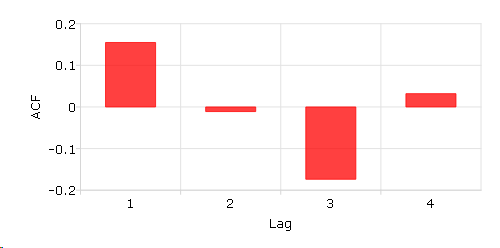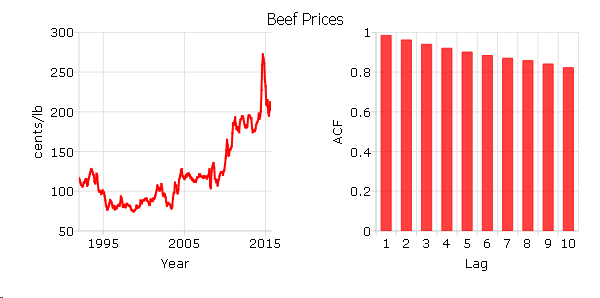# acf¶

## Purpose¶

Computes sample autocorrelations.

## Format¶

rk = acf(y, k, d)
Parameters:
• y (Nx1 vector) – univariate time series.

• k (scalar) – maximum number of autocorrelations to compute. 0 < k < N.

• d (scalar) – order of differencing. Set d equal to 0 to compute the autocorrelations from the original time series.

Returns:

rk (Kx1 vector) – sample autocorrelations.

## Examples¶

### Basic example¶

// Short time-series
x = { 12.92,
14.28,
13.31,
13.34,
12.71,
13.08,
11.86,
9.000,
8.190,
7.970,
8.350,
8.200,
8.120,
8.390,
8.660  };

// Maximum number of lags
k = 4;

// Order of differencing
d = 1;

// Calculate and print result of autocorrelation function
rk = acf(x, k, d);
print rk;


The code above produces the following output:

0.15488076
-0.011078336
-0.17367167
0.031921209


Plot the ACF results with plotBar() function. The first input 0 means using sequence numbers to name elements in the rk.

plotBar(0, rk);### Calculate the autocorrelation function and plot the results for “beef_prices” data.¶

// Get file name with full path
file = getGAUSSHome() \$+ "examples/beef_prices.csv";

// Import dataset starting with row 2 and column 2

// Max lags
k = 10;

// Order of differencing
d = 0;

// Call acf function
beef_acf = acf(beef, k, d);


The following code can give the time plot and sample ACF plot based on the beef and beef_acf:

/*
** Time series plot
*/

// Declare plotControl structure and
// fill with default values for XY plots
struct plotControl ctl;
ctl = plotGetDefaults("xy");

// Split canvas into a 1x2 grid.
// Place next graph in first cell.
plotLayout(1, 2, 1);

// Labels and format setting based on 'beef' time series
plotSetYLabel(&ctl, "cents/lb");
plotSetXLabel(&ctl, "Year");
plotSetXTicLabel(&ctl, "YYYY");
plotSetXTicInterval(&ctl, 120, 199501);

// Draw time series plot
plotTS(ctl, 1992, 12, beef);

// Split canvas into a 1x2 grid.
// Place next graph in second cell.
plotLayout(1, 2, 2);

/*
** ACF plot
*/

// Fill 'ctl' structure with bar plot defaults
ctl = plotGetDefaults("bar");

// Set labels and format based on 'beef_acf' matrix
plotSetYLabel(&ctl, "ACF");
plotSetXLabel(&ctl, "Lag");

// Draw ACF plot
plotBar(ctl, seqa(1, 1, k), beef_acf);


The above code will create the followig plot:## Source¶

tsutil.src

Functions pacf()